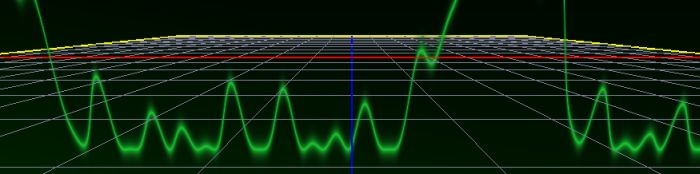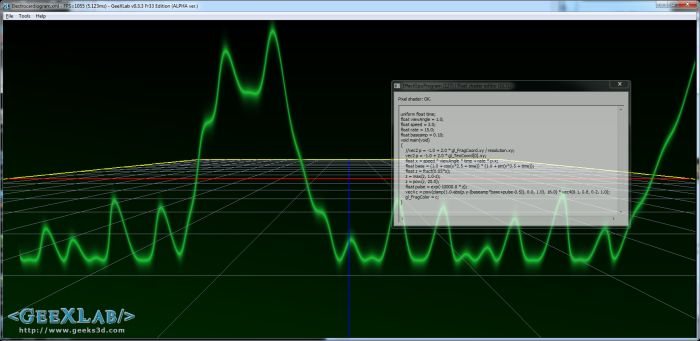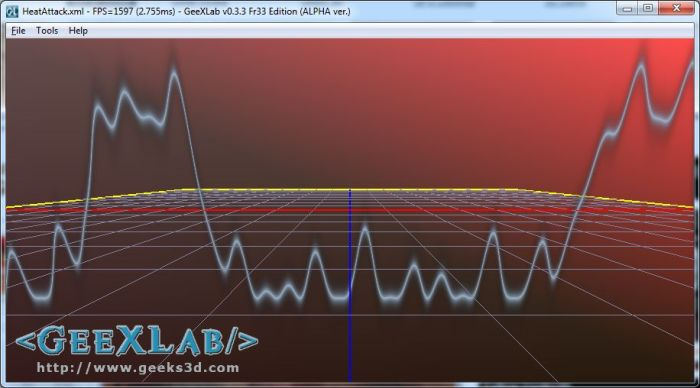# (Shader Library) Electrocardiogram Effect (GLSL)Here is a cool GLSL effect that simulates an electrocardiogram (ECG). The original effect, in HLSL, comes from the book Hackish C++ Games and Demos (by Michael Flenov). All work is done in the pixel shader.

Here is the complete GLSL program for GeeXLab (easily portable to any other GLSL-based app):

```[Vertex_Shader]
#version 120
void main()
{
gl_TexCoord = gl_MultiTexCoord0;
gl_Position = ftransform();
}

uniform float time;
float viewAngle = 1.0;
float speed = 3.0;
float rate = 15.0;
float baseamp = 0.10;
void main(void)
{
vec2 p = -1.0 + 2.0 * gl_TexCoord.xy;
float x = speed * viewAngle * time + rate * p.x;
float base = (1.0 + cos(x*2.5 + time)) * (1.0 + sin(x*3.5 + time));
float z = fract(0.05*x);
z = max(z, 1.0-z);
z = pow(z, 20.0);
float pulse = exp(-10000.0 * z);
vec4 ecg_color = vec4(0.6, 0.7, 0.8, 1.0);
vec4 c = pow(clamp(1.0-abs(p.y-(baseamp*base+pulse-0.5)), 0.0, 1.0), 16.0) * ecg_color;
gl_FragColor = c;
}
```